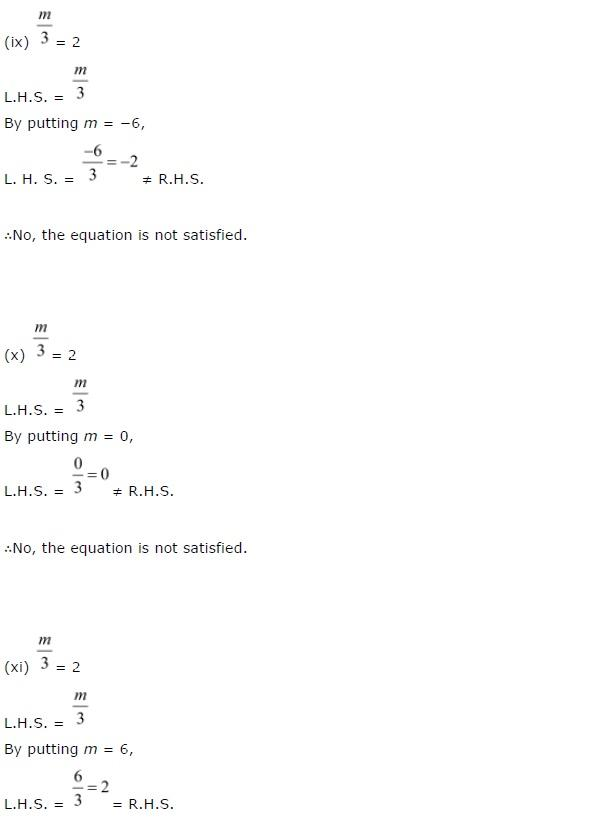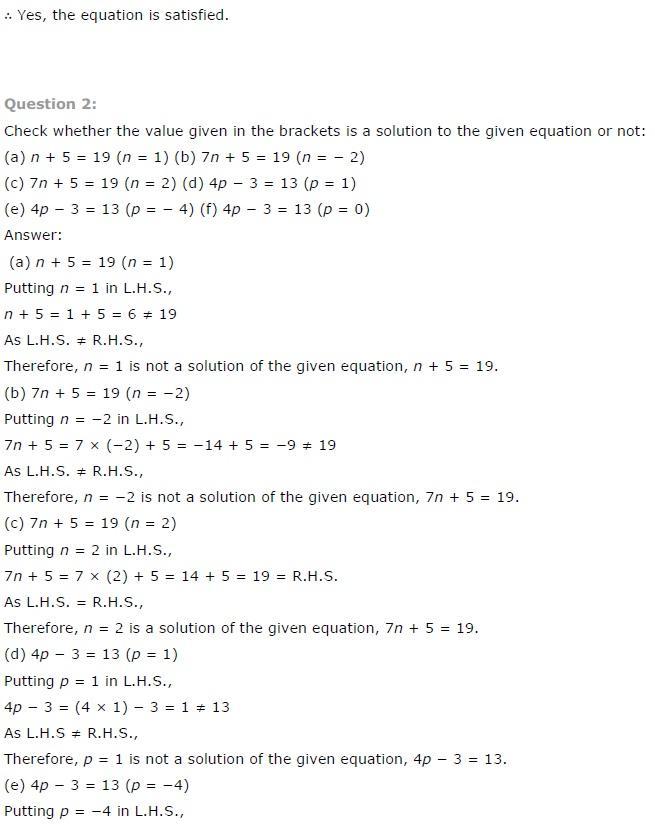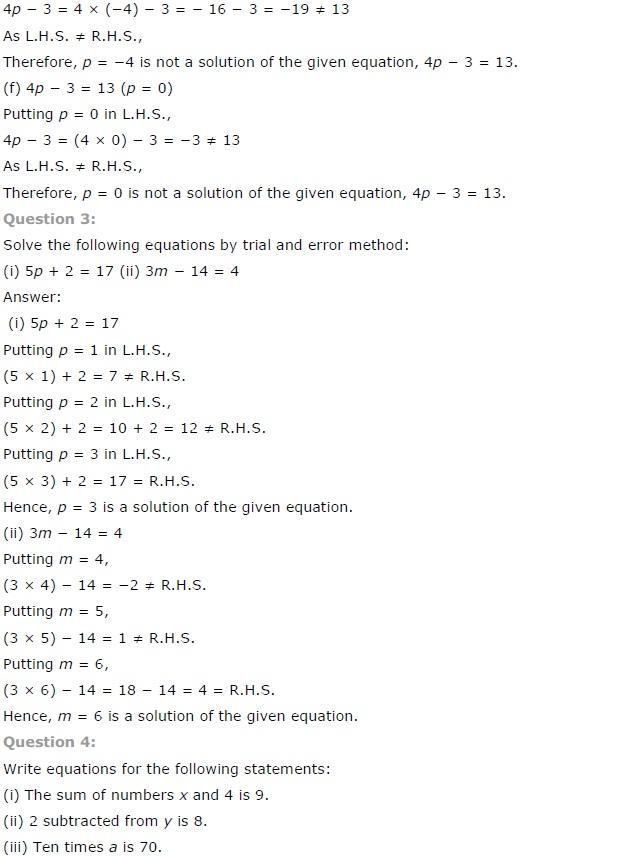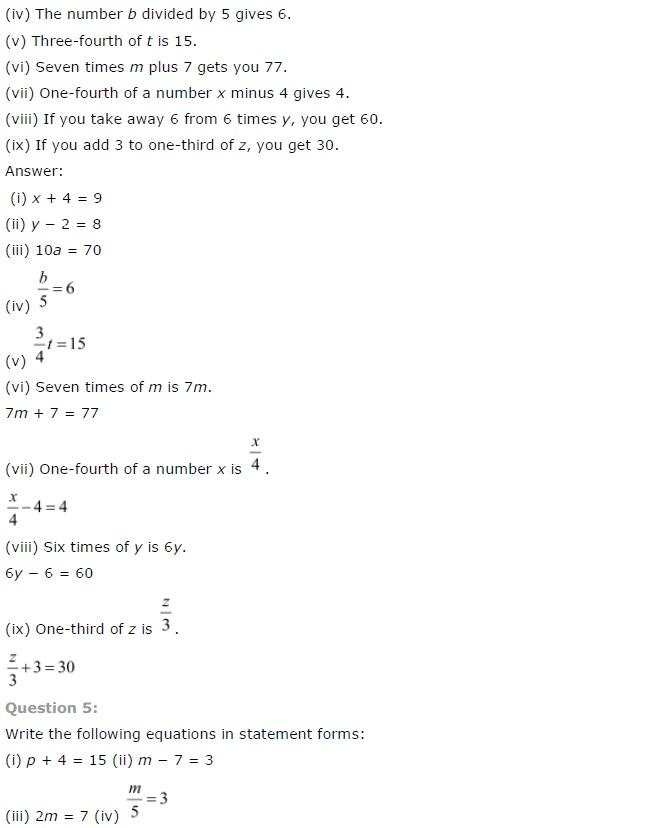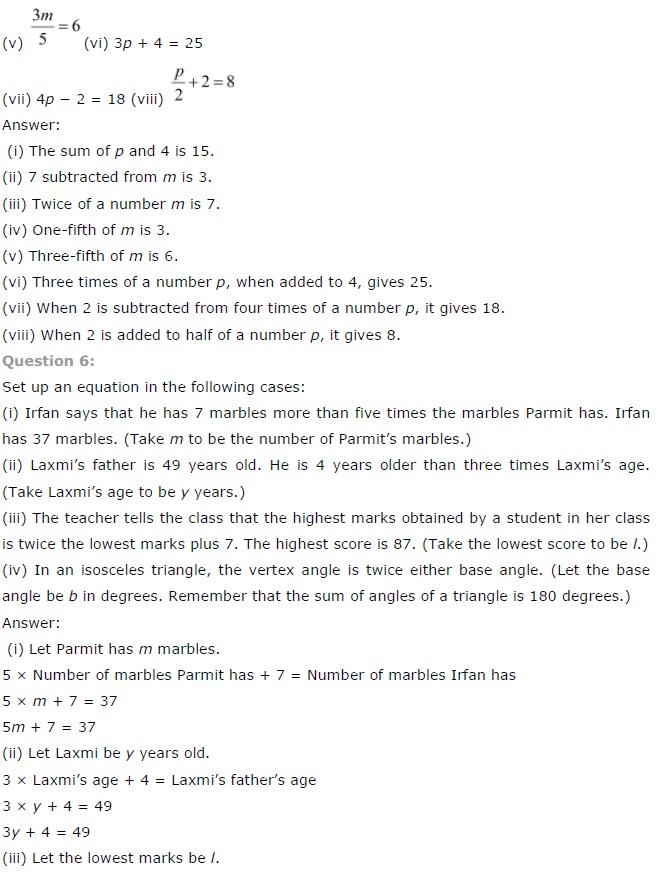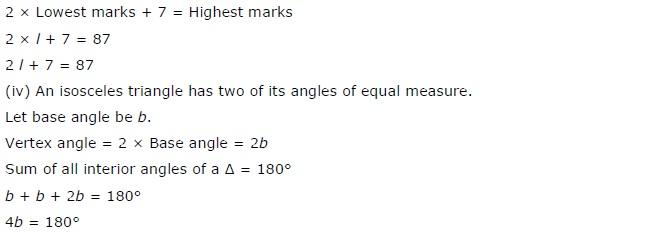# NCERT Solutions class 7 Maths Chapter-4 Exercise 4.1

NCERT Solutions Class-7 Maths chapter-4 Linear equation Exercise-4.1 is prepared by academic team of Physics Wallah all the questions of NCERT text book are solved step by step with proper and detail solutions explaining each and every questions. For More and additional questions of CBSE class 7 maths you can go to class 7 maths sections. NCERT class 7 Maths Solutions is the best way to enhanced your mathematics skill. And Physics Wallah practice worksheet & question bank will help you a lot .

## NCERT Solutions class 7 Maths Chapter-4 Linear equation

### Solutions of Chapter Linear equation  Exercise-4.1

#### Question 1:

Complete the last column of the table.

 S.no. Equation Value Say, whether the equation is satisfied. (yes/No) (i) x + 3 = 0 x = 3 (ii) x + 3 = 0 x = 0 (iii) x + 3 = 0 x = -3 (iv) x - 7 = 1 x = 7 (v) x - 7 = 1 x = 8 (vi) 5x = 25 x = 0 (vii) 5x = 25 x = 5 (viii) 5x = 25 x = -5 (ix) m/3 = 2 m = -6 (x) m/3 = 2 m = 0 (xi) m/3 = 2 m = 6

(i) The equation we have is: x + 3 = 0

Note: To check whether x =3 satisfies the given equation, we put x = 3 in the equation.

L.H.S. = 3 + 3 = 6 ≠ RHS

Thus, the answer is No i.e. the equation is not satisfied.

(ii) The equation we have is: x + 3 = 0

Note: To check whether the x = 0 satisfies the given equation, we put x = 0 in the given equation.

L.H.S. = 0 + 3 = 3 ≠ RHS

Thus, the answer is No i.e. the equation is not satisfied.

(iii) The equation we have is: x + 3 = 0
Note: To check whether the x = -3 satisfies the given equation, we put x = -3 in the given equation.
L.H.S. = -3 + 3 = 0 = RHS
Thus, the answer is Yes i.e. the equation is satisfied.

(iv) The equation we have is: x – 7 = 1
Note: To check whether the x = 7 satisfies the given equation, we put x = 7 in the given equation.
L.H.S. = 7 – 7 = 0 ≠ RHS
Thus, the answer is No i.e. the equation is not satisfied.

(v) The equation we have is: x – 7 = 1
Note: To check whether the x = 8 satisfies the given equation, we put x = 8 in the given equation.
L.H.S. = 8 – 7 = 1 = RHS
Thus, the answer is Yes i.e. the equation is satisfied.

(vi) The equation we have is: 5x = 25
Note: To check whether the x = 0 satisfies the given equation, we put x = 0 in the given equation.
L.H.S. = 5(0) = 0 ≠ RHS
Thus, the answer is No i.e. the equation is not satisfied.

(vii) The equation we have is: 5x = 25
Note: To check whether the x = 5 satisfies the given equation, we put x = 5 in the given equation.
L.H.S. = 5(5) = 25 = RHS
Thus, the answer is Yes i.e. the equation is satisfied.

(viii) The equation we have is: 5x = 25
Note: To check whether the x = -5 satisfies the given equation, we put x = -25 in the given equation.
L.H.S. = 5(-5) = -25 = RHS
Thus, the answer is No i.e. the equation is not satisfied.